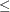### Flow and Value Bounds

The capacity and lower flow bound of an arc can be equal. Negative arc capacities and lower flow bounds are permitted. If both arc capacities and lower flow bounds are negative, the lower flow bound must be at least as negative as the capacity. An arc (A,B) that has a negative flow ofunits can be interpreted as an arc that conveysunits of flow from node B to node A.

The upper and lower value bound of a nonarc variable can be equal. Negative upper and lower bounds are permitted. If both are negative, the lower bound must be at least as negative as the upper bound.

When solving an LP, the upper and lower value bounds of an LP variable can be equal. Negative upper and lower bounds are permitted. If both are negative, the lower bound must be at least as negative as the upper bound.

In short, for any problem to be feasible, a lower bound must bethe associated upper bound.